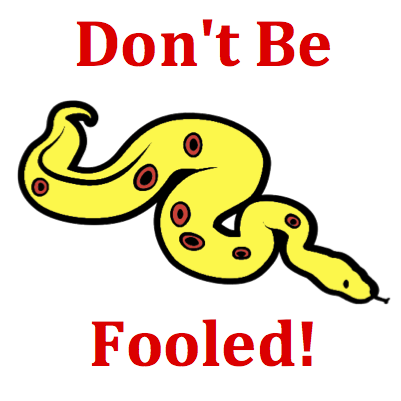# Momentum and Collisions - Mission MC5 Detailed HelpIn a Physics lab, two carts of identical mass collide on a low-friction track in such a manner that the system can be considered as an isolated system. The before- and after-collision velocities of the carts are represented by vector arrows. Which vector represents the after-collision velocity of cart B?The momentum of the carts is the product of mass times velocity (see Formula Frenzy section). The mass is listed as m or 2m or ... ; think of it as 1 kg, 2 kg or ... if it makes it easier for you. The velocity can be determined by measuring the length of the vector arrow; use the on screen ruler to do this. The product of the mass and velocity tells you the momentum of that cart. By adding together cart A's and B's momentum before the collision, the total amount of system momentum can be determined. Since the collision is said to be conserved in an isolated system, the total amount of momentum after the collision should be the same as before. (See Know the Law - The Law of Momentum Conservation section.) Object A's velocity can be measured for the after collision diagram and its momentum can be determined. The after-collision momentum of cart B must make up the difference between cart A's after-collision momentum and the total amount of momentum. Determine the momentum value of cart B; then divide by the mass to determine the velocity of cart B. Then pick the appropriate choice from among the available possibilities.The Law of Momentum Conservation: If a collision occurs between object 1 and object 2 in an isolated system, then the momentum change of object 1 is equal in magnitude and opposite in direction to the momentum change of object 2. In equation form m1 • ∆v1 = - m2 • ∆v2   The total momentum of the system before the collision (p1 + p2) is the same as the total momentum of the system of two objects after the collision (p1' + p2'). That is   p1 + p2 = p1' + p2' Total system momentum is said to be conserved for any collision occurring in an isolated system.Momentum as a Vector: Momentum is a vector and it has a direction. The direction of an object's momentum is in the same direction that the object is moving.A common student mistake is to think that the quantity that is conserved in a collision is velocity. But don't be fooled! The sum of the velocities before the collision is NOT necessarily equal to the sum of the velocities after the collision. Momentum is the quantity that is being conserved, not velocity. The velocity values must be multiplied by the listed mass values in order to determine the momentum. Then apply the law of momentum conservation (see Know the Law - The Law of Momentum Conservation section).The diagram shows momentum vectors for objects A and B before and after the collision. The length of the vector arrow represents the amount of momentum the object possesses. There are grid marks on each vector that can be used to measure its length. Once measured, you would be wise to write it down on some scratch paper. You'll need most your active mind for processing; don't waste space for memory purposes.The momentum (p) of an object can be calculated from its mass (m) and velocity (v) using the formula:       p = m • v